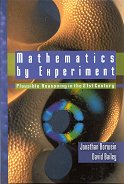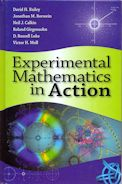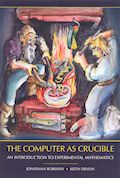## Books : reviews### Jonathan M. Borwein, David H. Bailey.Mathematics by Experiment: plausible reasoning in the 21st century. A K Peters. 2004

Mathematicians have always used experiments and visualization to explore new ideas and ways to prove them.

Using examples that truly represent the experimental methodology, this book provides the historical context of, and rationale behind, experimental mathematics. It shows how today, the use of advanced computing technology provides mathematicians with an amazing, previously unimaginable “laboratory,” in which examples can be analyzed, new ideas tested, and patterns discovered.### David H. Bailey, Jonathan M. Borwein, Neil J. Calkin, Roland Girgensohn, D. Russell Luke, Victor H. Moll.Experimental Mathematics in Action. A K Peters. 2007

The last twenty years have been witness to a fundamental shift in the way mathematics is practiced. With the continued advance of computing power and accessibility, the view that “ real mathematicians don’t compute” no longer has any traction for a newer generation of mathematicians that can really take advantage of computer-aided research, especially given the scope and availability of modern computational packages such as Maple, Mathematica, and MATLAB. The authors provide a coherent variety of accessible examples of modern mathematics subjects in which intelligent computing plays a significant role.### Jonathan M. Borwein, Keith Devlin.The Computer as Crucible: an introduction to experimental mathematics. A K Peters. 2009

rating : 4 : passes the time
review : 19 September 2016

For a long time, pencil and paper were considered the only tools needed by a mathematician (some might add the waste basket). As in many other areas, computers play an increasingly important role in mathematics and have vastly expanded and legitimized the role of experimentation in mathematics. How can a mathematician use a computer as a tool? What about as more than just a tool, but as a collaborator?

Keith Devlin and Jonathan Borwein, two well-known mathematicians with expertise in different mathematical specialities but with a common interest in experimentation in mathematics, have joined forces to create this introduction to experimental mathematics. They cover a variety of topics and examples to give the reader a good sense of the current state of play in the rapidly growing new field of experimental mathematics.

The discipline of mathematics has many aspects. There is the process of exploration, “messing around”, spotting patterns, suggesting hypotheses and conjectures. Then there is the process of proof: going from the initial statement to the fully proven theorem, which in non-trivial cases will also involve significant elements of exploration. Finally, there is the theorem itself, a statement of mathematical fact, backed up by a rock-solid argument of the tidied-up proof. Often though, only that final proof, and none of the processes leading up to it, is made public. This can lead to an external view of mathematics as magical results of genius, rather than a process of creative hard graft.

In my discipline of computer science, there is some support for the process of proof, with a computer helping to make sure the steps really are rock solid, and not instead built on sand. Unfortunately, the computer is a super-pedant, even more so that the most nit-picking of mathematicians, and nothing but the most adamantine of rock will do for it. Valid short cuts such as “without loss of generality”, “by symmetry”, and “abusing the notation” are not allowed, making these tools somewhat exasperating to use at times (although they are improving all the time).

But what about that initial process of exploration? Experimental Mathematics augments that stage: using a computer to help “messing around”, taking away (some of) the tedium of exploratory calculations, providing more examples and help with pattern discovery.

This book provides an introduction to such Experimental Mathematics, with several examples, and suggested exercises if you feel inclined to pursue some of the ideas further. Many of the examples are of the form:

1. Have a mathematical expression such as a series formula, or definite integral, from somewhere (maybe a previous round of exploration)
2. Use a computer to get a good numerical approximation to the expression, eg, 3.1462643699419723423
3. Use a program to suggest a possible closed form representation of that number, eg, in this case, √2 + √3
4. Use that closed form as a conjecture for the actual value of the expression, and try to prove it (which may include further experimental mathematics)

There are several examples of increasing complexity, covering sequences, series, integrals, and more, with discussion of the discoveries of the results and their proofs. There is discussion about the famous formula that allows the calculation of the nth binary digit of π, without needing to calculate all the preceding n–1 digits. I also learned of “spigot” algorithms to calculate numerical values, which produce the digits one by one, cutting down on memory requirements.

The book definitely gives a flavour of the overall process, with enough pointers to suitable software resources for the reader to take up some of the challenges. However, I was left feeling that the flavour was somewhat weak. Okay, now we can calculate π to a bazillion digits, or find its gazillionth (binary) digit without calculating the earlier ones, and we have proofs of lots of closed form solutions. And yet, I didn’t come away with a feeling of much mathematical depth in these results. At most, in some cases the process suggests links between seemingly unrelated expressions. But I was hoping for more significant results. For example, the book finishes with a short chapter on “discovery by visualisation”, an area where the computer surely reigns supreme, yet surprisingly little is made of this. Maybe I am simply unaware of a vast existing industry in mathematics that is about proving such seemingly-pedestrian but actually important results, which has now become much more automated and hence more productive?